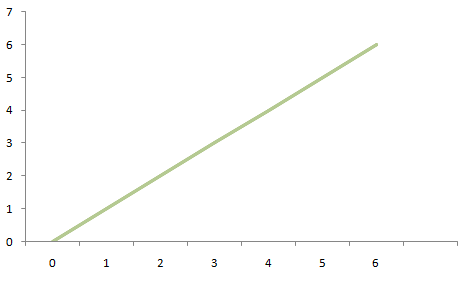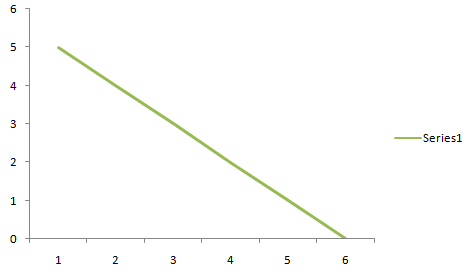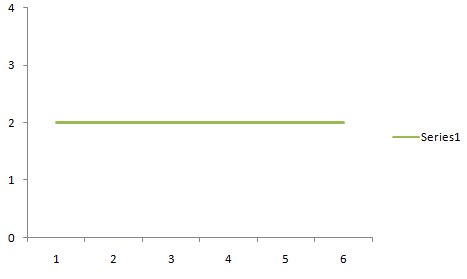# Correlation Coefficient Formula

• Last Updated : 16 Feb, 2022

Correlation coefficients are used to calculate how vital a connection is between two variables. There are different types of correlation coefficients, one of the most popular is Pearson’s correlation (also known as Pearson’s R)which is commonly used in linear regression.

### Correlation coefficient Formula

The correlation coefficient procedure is used to determine how strong a relationship is between the data. The correlation coefficient procedure yields a value between 1 and -1. In which,

• -1 indicates a strong negative relationship
• 1 indicates strong positive relationships
• And an outcome of zero implies no connection at allPositive CorrelationNegative CorrelationZero Correlation

Meaning

• A correlation coefficient of -1 means there is a negative decrease of a fixed proportion, for every positive increase in one variable. Like, the amount of gas in a tank decreases in a perfect correlation with the speed.
• A correlation coefficient of  1 means there is a positive increase of a fixed proportion of others, for every positive increase in one variable. Like, the size of the shoe goes up in perfect correlation with foot length.
• Zero means that for every increase, there is neither a positive nor a negative increase. The two just aren’t related.

### Types of Formulae

• Pearson’s correlation coefficient formula• Sample correlation coefficient formulaSxy is the sample Covariance, and Sx and Sy are the sample standard deviations

• Population correlation coefficient formulaIt uses σx and σy as the population standard deviation and, σxy as the population Covariance.

### Pearson’s correlation

It is the most common correlation in statistics. The full name is Pearson’s Product Moment correlation in short PPMC. It displays the Linear relation between the two sets of data. Two letters are used to represent the Pearson correlation: the Greek letter rho (ρ) for a population and the letter “r” for a sample correlation coefficient.

Steps to find Pearson’s correlation coefficient

Step 1: Firstly make a chart with the given data like subject,x, and y and add three more columns in it xy, x² and y².

Step 2: Now multiply the x and y columns to fill the xy column. For example:- in x we have 24 and in y we have 65 so xy will be 24×65=1560.

Step 3: Now, take the square of the numbers in the x column and fill the x² column.

Step 4: Now, take the square of the numbers in the y column and fill the y² column.

Step 5: Now, add up all the values in the columns and put the result at the bottom. Greek letter sigma (Σ) is the short way of saying summation.

Step 6: Now, use the formula for Pearson’s correlation coefficient:To know which type of variable we have either positive or negative.

### Linear Correlation Coefficient

The Pearson’s correlation coefficient is the linear correlation coefficient which returns the value between the -1 and +1. In this -1 indicates a strong negative correlation and +1 indicates a strong positive correlation. If it lies 0 then there is no correlation. This is also known as zero correlation.

The “crude estimations” for analyzing the stability of correlations using Pearson’s Correlation:

### Cramer’s V Correlation

It is as similar as the Pearson correlation coefficient. It is used to calculate the correlation with more than 2×2 rows and columns. Cramer’s V correlation varies between 0 and 1. The value close to zero associates that a very little association is there between the variables and if it’s close to 1 it indicates a very strong association.

The “crude estimates” for interpreting strengths of correlations using Cramer’s V Correlation:

### Sample Problems

Problem 1: Calculate the correlation coefficient from the following table:

Solution:

Make a table from the given data and add three more columns of XY, X², and Y².

∑xy = 20379

∑x = 244

∑y = 488

∑x² = 11086

∑y² = 40266

n = 6.

Put all the values in the Pearson’s correlation coefficient formula:R = 6(20379) – (244)(488) / √[6(11086)-(244)²][6(40266)-(488)² ]

R = 3202 / √

R = 3202/4972.238

R = 0.6439

It shows that the relationship between the variables of the data is a strong positive relationship.

Problem 2: Calculate the correlation coefficient from the following table:

Solution:

Make a table from the given data and add three more columns of XY,  X², and Y².

∑xy = 12258

∑x = 151

∑y = 336

∑x² = 5625

∑y² 28724

n = 4

Put all the values in the Pearson’s correlation coefficient formula:R = 4(12258) – (151)(336) / √[4(5625)-(151)²][4(28724)-(336)²]

R = -1704 / √[-301][-2000]

R=-1704/775.886

R=-2.1961

It shows that the relationship between the variables of the data is a very strong negative relationship.

Problem 3:  Calculate the correlation coefficient for the following data:

X = 7,9,14 and Y = 17,19,21

Solution:

Given variables are,

X = 7,9,14

and,

Y = 17,19,21

To, find the correlation coefficient of the following variables Firstly a table is to be constructed as follows, to get the values required in the formula.

∑xy = 584

∑x = 30

∑y = 57

∑x² = 326

∑y² = 838

n = 3

Put all the values in the Pearson’s correlation coefficient formula:R = 3(584) – (30)(57) / √[3(326)-(30)²][3(838)-(57)²]

R = 42 / √[-735]

R = 42/-239.43

R = -0.1754

It shows that the relationship between the variables of the data is negligible relationship

Problem 4: Calculate the correlation coefficient for the following data:

X = 21, 31, 25, 40, 47, 38 and Y = 70,55,60,78,66,80

Solution:

Given variables are,

X = 21,31,25,40,47,38

And,

Y = 70,55,60,78,66,80

To, find the correlation coefficient of the following variables Firstly a table is to be constructed as follows, to get the values required in the formula.

∑xy = 13937

∑x = 202

∑y = 409

∑x² = 7280

∑y² = 28265

n = 6

Put all the values in the Pearson’s correlation coefficient formula:R = 6(13937) – (202)(409) / √[6(7280) – (202)²][6(28265) – (409)²]

R = 1004 /√

R = 1004 / 2892.452938

R = 0.3471

It shows that the relationship between the variables of the data is a moderate positive relationship.

Problem 5: Calculate the correlation coefficient for the following data?

X = 5 ,9 ,14, 16 and Y = 6, 10, 16, 20 .

Solution:

Given variables are,

X = 5 ,9 ,14, 16

And

Y = 6, 10, 16, 20.

To, find the correlation coefficient of the following variables Firstly a table is to be constructed as follows, to get the values required in the formula add all the values in the columns to get the values used in the formula

∑xy = 664

∑x = 44

∑y = 52

∑x² = 558

∑y² = 792

n = 4

Put all the values in the Pearson’s correlation coefficient formula:R = 4(664) – (44)(52) / √[4(558) – (44)²][4(792) – (52)²]

R = 368 / √

R = 368/370.599

R = 0.9930

It shows that the relationship between the variables of the data is a very strong positive relationship.

Problem 6: Calculate the correlation coefficient for the following data:

X = 10, 13, 15 ,17 ,19 and Y = 5,10,15,20,25.

Solution:

Given variables are,

X = 10, 13, 15 ,17 ,19 and Y = 5, 10, 15, 20, 25.

To, find the correlation coefficient of the following variables Firstly a table is to be constructed as follows, to get the values required in the formula also  add all the values in the columns to get the values used in formula,

∑xy = 1103

∑x = 74

∑y = 75

∑x² = 1144

∑y² = 1375

n = 5

Put all the values in the Pearson’s correlation coefficient formula:R = 5(1103) – (74)(75) / √ [5(1144) – (74)²][5(1375) – (75)²]

R = -35 / √

R = -35/552.26

R = 0.0633

It shows that the relationship between the variables of the data is a negligible relationship.

Problems 7: Calculate the correlation coefficient for the following data:

X = 12, 10, 42, 27,35,56 and Y = 13, 15, 56, 34,65,26

Solution:

Given variables are,

X = 12, 10, 42, 27, 35, 56 and Y = 13, 15, 56, 34, 65, 26

To, find the correlation coefficient of the following variables Firstly a table is to be constructed as follows, to get the values required in the formula also add all the values in the columns to get the values used in the formula

∑xy = 7307

∑x = 182

∑y = 209

∑x² = 7098

∑y² = 9587

n = 6

Put all the values in the Pearson’s correlation coefficient formula:R = 6(7307) – (182)(209) / √ {[6(7098) – (182)²][6(9587)-(209)²]}

R = 5804 / √

R = 5804/11445.139

R = 0.5071

It shows that the relationship between the variables of the data is a strong positive relationship.

My Personal Notes arrow_drop_up